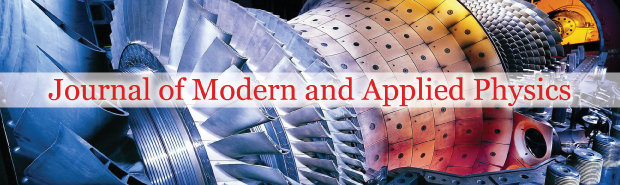All submissions of the EM system will be redirected to Online Manuscript Submission System. Authors are requested to submit articles directly to Online Manuscript Submission System of respective journal.### The d’Alembertian operator and Maxwell’s equations

Author(s): Claude Michael Cassano*

The d’Alembertian is a linear second order differential operator, typically in four independent variables. The time-independent version (in three independent (space) variables is called the Laplacian operator. When its action on a function or vector vanishes, the resulting equation is called the wave equation (or Laplace’s equation). When it’s action is identified with a non-zero function (or vector function) the resulting equation is called Poisson’s equation. This equation is fundamental to and of great importance in field theory. The focus of this tutorial is to demonstrate that when acting on a four-vector, the d’Alembertian may be factored into two 4 x 4 differential matrices. This d’Alembertian operator factorization of a four-vector into two 4 x 4 differential matrices is not merely another form of expressing Maxwell’s equations; but remarkably, yields a quantum and unified field theory generalization.

Full-Text | PDF

## Recommended Conferences

### International Conference on Materials Physics and Materials Science

Tokyo, JapanTop